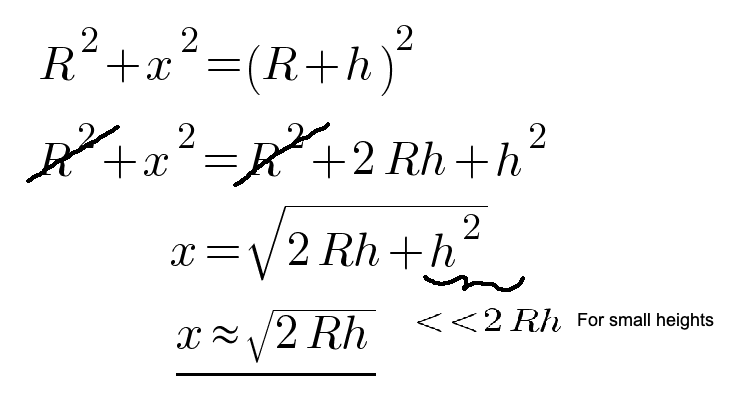How Far Is the Horizon

You can see a staggering 200+ miles from a commercial airplane

The distance to the horizon depends on how high you are observing it.

If you are 5'6'’, standing on the shoreline, the horizon appears 2.75 miles away from you.

In this article, you learn how to calculate the distance to the horizon with a simple formula.

How Far Can You See From a Plane

Commercial aircraft fly between 9.5km — 11.6km (31 000ft — 38000ft).

This means you can see 350km — 390km (215–238 miles) from an airplane.

How Far Can You See From the Top of Mount Everest

Mount Everest is 8849 meters (23901 feet) high.

This means you can see 335 km (209 miles) from the top of it. Of course, this assumes there are no other mountains blocking the view to the horizon.

How Far Can You See From Burj Khalifa

Burj Khalifa is the tallest building in the world. It is 829.8 meters (2722 feet) high.

This means you can see 103 km (64 miles) away from the top of it.

How to Calculate the Distance to Horizon

Here is one meter-based and one feet-based formula to easily calculate the distance to the horizon.

Feet Formula

Given the observation height in feet, you can get the distance to the horizon in miles with this formula:

Meters Formula

Given the observation height in meters, you can get the distance to the horizon in kilometers with this formula:

Deriving the Equation for the Distance to Horizon

This is for someone who wants to know how to derive these formulas.

To derive the equation for how far is the horizon, use basic trigonometry. Namely, the Pythagorean theorem can be used to determine the distance to the horizon.

This figure illustrates the setup:

This setup forms a right triangle (the angle between R and x is 90 degrees). In other words, you can use the Pythagorean theorem:This approximation works well when dealing with heights far less than the radius of the earth (6370km).

This formula takes the height in meters and returns the distance in meters.

But this assumes you remember what is the radius of the earth (6370km). Furthermore, not everybody knows meters.

Let’s simplify this further for the non-math oriented.

Distance in Miles Given Height in Feet

Let’s create a simple version of the distance-to-horizon formula that works with feet and miles.

Notice this term:

Is a constant (earth's radius R times two). If you compute a numeric value for it, you get:

This simplifies the formula to:

Where x and h are in meters.

If you want to give h in feet and get the result in miles, divide the whole thing by one mile (1609 meters) and factor out the meters to feet coefficient (1/0.3048):

This is the formula you saw earlier:

Distance in Kilometers Given Height in Meters

This formula is easy to derive—divide the result by 1000, and simplify with approximations:

For example, to calculate the distance in miles given the

Conclusion

The distance to the horizon can be calculated with basic trigonometry.

To know the distance in kilometers given height in meters, use this formula:

To know the distance in miles given height in feet, use this formula:

Thanks for reading. I hope you enjoy it!